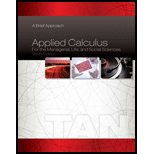Chapter 2.2, Problem 67E### Applied Calculus for the Manageria...

10th Edition
Soo T. Tan
ISBN: 9781285464640

#### Solutions

Chapter
Section### Applied Calculus for the Manageria...

10th Edition
Soo T. Tan
ISBN: 9781285464640
Textbook Problem

# Family Insurance Coverage The average annual worker and employer contributions (in dollars) to premiums for family insurance coverage from 2005 (t = 1) through 2011 (t = 7) are approximated by the functions.f(t) = 4.3809t3 − 47.833t2 + 374.49t + 2390 (1 ≤ t ≤7)andg(t) = 13.222t3 − 132.524t2 + 757.9t + 7491 (1 ≤ t ≤7)respectively. a. Find a function h giving the total premiums for family coverage. b. Find the average annual worker contribution to premiums in 2010 (t = 6). the employer’s contribution to premiums in 2010, and the total contributions to premiums. c. Compute h(6). Compare the result with the total contributions to premiums in 2010 obtained in part (b). Source: Kaiser/HRET Survey of Employer-Sponsored Health Benefits.

(a)

To determine

To find: The function h which gives the total premiums for family coverage.

Explanation

Given information:

The functions for average annual worker and employer contribution to premiums for family coverage from 2005 to 2011 are,

f(t)=4.389t347.833t2+374.49t+2390

And

g(t)=13.222t3132.524t2+757.9t+7481

Calculation:

The function h is the sum of the functions f(t) and g(t) .

h(t)=f(t)+g(t) (1)

Substitute 4.389t347.833t2+374.49t+2390 for f(t) and 13.222t3132.524t2+757.9t+7481 for g(t) in equation (1).

h(t)=[(4

(b)

To determine

To find: The average annual worker and employer contribution to premiums in 2010 at t=6 and total contribution to premiums.

(c)

To determine

To compute: The value of h(6) and to compare the result with total contributions to premiums obtained in part (b).

### Still sussing out bartleby?

Check out a sample textbook solution.

See a sample solution

#### The Answers to Your Study Problems

Solve them all with bartleby. Boost your grades with guidance from subject experts covering thousands of textbooks. All for just \$9.99/month

Get As ASAP

## Additional Math Solutions

#### Find more solutions based on key concepts